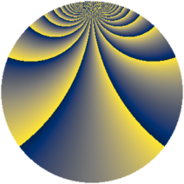# Properties

 Label 1045.2.ckLevel $1045$ Weight $2$ Character orbit 1045.ck Rep. character $\chi_{1045}(4,\cdot)$ Character field $\Q(\zeta_{90})$ Dimension $2784$ Sturm bound $240$

# Related objects

## Defining parameters

 Level: $$N$$ $$=$$ $$1045 = 5 \cdot 11 \cdot 19$$ Weight: $$k$$ $$=$$ $$2$$ Character orbit: $$[\chi]$$ $$=$$ 1045.ck (of order $$90$$ and degree $$24$$) Character conductor: $$\operatorname{cond}(\chi)$$ $$=$$ $$1045$$ Character field: $$\Q(\zeta_{90})$$ Sturm bound: $$240$$

## Dimensions

The following table gives the dimensions of various subspaces of $$M_{2}(1045, [\chi])$$.

Total New Old
Modular forms 2976 2976 0
Cusp forms 2784 2784 0
Eisenstein series 192 192 0

## Trace form

 $$2784 q - 36 q^{4} - 18 q^{5} - 36 q^{6} - 36 q^{9} + O(q^{10})$$ $$2784 q - 36 q^{4} - 18 q^{5} - 36 q^{6} - 36 q^{9} - 48 q^{10} - 18 q^{11} + 24 q^{14} - 42 q^{15} - 12 q^{16} - 36 q^{19} + 6 q^{20} - 60 q^{21} - 36 q^{24} + 54 q^{25} - 54 q^{26} - 36 q^{29} - 9 q^{30} - 18 q^{31} - 96 q^{34} + 12 q^{35} - 138 q^{36} - 72 q^{39} + 84 q^{40} + 18 q^{41} - 54 q^{44} - 30 q^{45} - 18 q^{46} - 204 q^{49} + 39 q^{50} - 108 q^{51} - 216 q^{54} - 150 q^{55} - 24 q^{56} - 144 q^{59} - 15 q^{60} - 132 q^{61} - 282 q^{64} - 24 q^{65} + 240 q^{66} - 18 q^{69} + 3 q^{70} - 60 q^{71} - 72 q^{74} - 36 q^{75} - 204 q^{76} - 36 q^{79} - 30 q^{80} - 192 q^{81} - 486 q^{84} - 210 q^{85} + 24 q^{86} - 168 q^{89} - 6 q^{90} + 6 q^{91} + 192 q^{94} - 132 q^{95} - 552 q^{96} - 144 q^{99} + O(q^{100})$$

## Decomposition of $$S_{2}^{\mathrm{new}}(1045, [\chi])$$ into newform subspaces

The newforms in this space have not yet been added to the LMFDB.